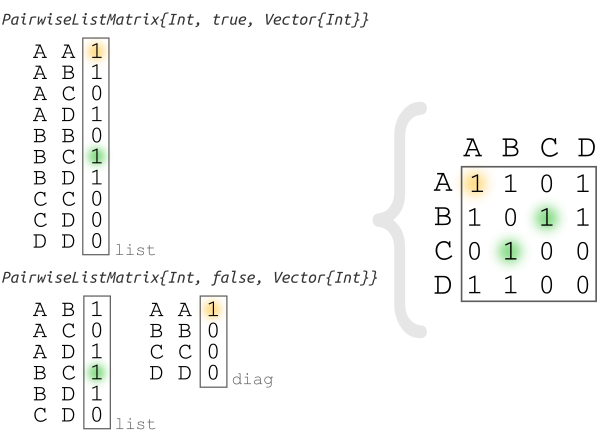dummy-link

13

0

9

7

# PairwiseListMatrices

## Description

This package allows you to use a pairwise list as a matrix:``````mutable struct PairwiseListMatrix{T,diagonal,VT} <: AbstractArray{T, 2}
list::VT
diag::VT
nelements::Int
...
end
``````

`PairwiseListMatrix{T, diagonal, VT}` is a (squared) symmetric matrix that stores a `list` of type `VT` with values of type `T` for the pairwise comparison/evaluation of `nelements`. If `diagonal` is `true` the first element of the list is `1, 1` otherwise is `1, 2`. If `diagonal` is `false` the diagonal values are stored in a vector on the `diag` field.

## Features

#### Space

In pairwise calculations like `cor()` if results are saved as `PairwiseListMatrix` the space is `N(N+1)/2` instead of `N*N`. This is useful to compare a large number of elements, because you are saving ~ 50% of the memory.

#### Time

`PairwiseListMatrix` is faster than a full matrix to make operatation like `sum` and `mean` in the whole matrix, since it is cache efficient. However it is slower than a full matrix for reducing along dimensions.

## Example

``````julia> # Pkg.add("PairwiseListMatrices")

julia> using PairwiseListMatrices

julia> plm  = PairwiseListMatrix([1,2,3], false)
3×3 PairwiseListMatrices.PairwiseListMatrix{Int64,false,Array{Int64,1}}:
0  1  2
1  0  3
2  3  0

julia> nplm  = setlabels(plm, ["a","b","c"])
3×3 Named PairwiseListMatrices.PairwiseListMatrix{Int64,false,Array{Int64,1}}
A ╲ B │ a  b  c
──────┼────────
a     │ 0  1  2
b     │ 1  0  3
c     │ 2  3  0

julia> table = to_table(nplm)
6×3 Matrix{Any}:
"a"  "a"  0
"a"  "b"  1
"a"  "c"  2
"b"  "b"  0
"b"  "c"  3
"c"  "c"  0

julia> from_table(table, true)
3×3 Named PairwiseListMatrix{Any, true, Vector{Any}}
A ╲ B │ a  b  c
──────┼────────
a     │ 0  1  2
b     │ 1  0  3
c     │ 2  3  0
``````

09/13/2015

23 days ago

145 commits